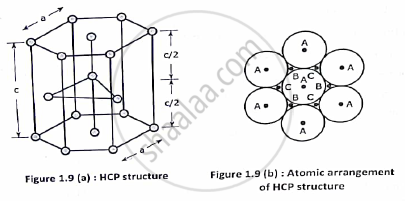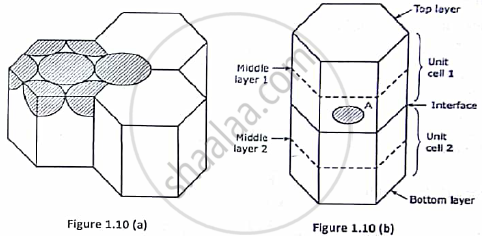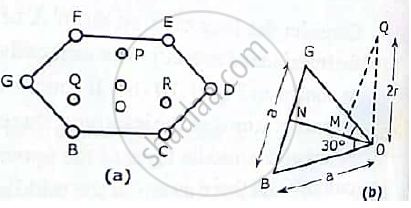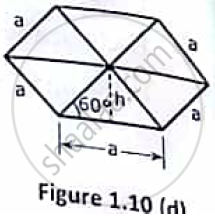Advertisement Remove all ads

# With neat diagram of unit cell , explain the structure of HCP crystal and calculate the no. of ions per unit cell, co ordination no., lattice constant and packing factor of the structure. - Applied Physics 1

Answer in Brief

With neat diagram of unit cell , explain the structure of HCP crystal and calculate the no. of ions per unit cell, co ordination no., lattice constant and packing factor of the structure.

Advertisement Remove all ads

#### Solution

This is the most common metallic structure exhibited by about twenty five metals, this structure has atomic arrangement in three layers as shown :-

STRUCTURE:-⦁ In the bottom layer the central atom is surrounded by six other identical atoms. The positions of these seven atoms are marked by A.
⦁ In the middle layer at height c/2 three atoms are positioned either at point B or at point C.
⦁ In the top layer at height C atomic distribution is similar to that of the bottom layer i.e., at point A.
NUMBER OF ATOMS/UNIT CELLS:-
Each corner atom is shared by 6 neighbouring unit cells. Hence each corner carriers (1/6)th of an atom as shown:-

Each face centre carriers ½ atom. In the middle layer there are three atoms. Hence total number of atoms/unit cells are

n = 2 (6×1/6) + 2(1/2) + 3 = 6COORDINATION NUMBER:-
Consider the face centred atom A of the interface of two adjacent unit cells. It touches six corner atoms of the interface three atoms of the middle layer of the upper unit cell and three atoms of the middle layer of the lower unit cell .
Hence , coordination number = 3(of middle layer 1) + 6(of the interface)+ 3(of middle layer 2). Therefore co-ordination number of HCP structure = 12.

ATOMIC PACKING FRACTION:-
1. Calculation of c/a :-
Consider the bottom layer of the unit cell shown. The atom are marked as B, C, D, E, F, G and O. the middle layer at height. c/2 consists of atoms marked as P, Q, and R. where a triangle is formed by joining O, B and G. this is an equilateral triangle of side a .Consider the middle layer atom Q which touches the face centred atom O. hence , OQ = 2r.

Draw a perpendicular QM from Q on ON. OM is the height of Q from the bottom layer. Hence = c/2 Since M is the orthocentre of ∆ OBG.

OM = 2/ 3 ON = 2/3 acos30° = 2/3 a(√3) / 2 = a/(√3 )

In ∆ OQM   OQ^2 = QM^2 + OM^2

(2r)^2 = ( c/2 )^ 2 + ( a /(√3)) ^2
As r = a/2 . This can be written as

Therefore ,  a^2 = ( c/2 )^2 + ( a/(√3) )^2

Hence c/a =√8 /3APF = ( n×4/3×π×r^3)/("volume of the unit cell")

As seen in figure, area of the hexagonal face = 6 × area of equilateral triangle

= 6 × 1/2 ah    = 3a ×a sin60°

= (3√3)/ 2a^2
Volume of the unit cell = Area × Height = (3√3)/2a^2c
For a HCP unit cell c/a =√ 8/3

Hence volume of the unit cell = (3√3)/2 a^3 c/a = 3√2a^3

Therefore APF =(6xx(4/ 3)π(a/2)^3)/(3√2a^3) = (√2)/6 π = 0.74

Concept: Study of characteristics of unit cell of Diamond, ZnS, NaCl and HCP;
Is there an error in this question or solution?
Advertisement Remove all ads

#### APPEARS IN

Advertisement Remove all ads
Advertisement Remove all ads
Share
Notifications

View all notifications

Forgot password?